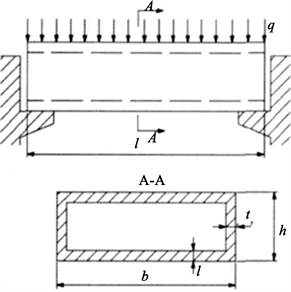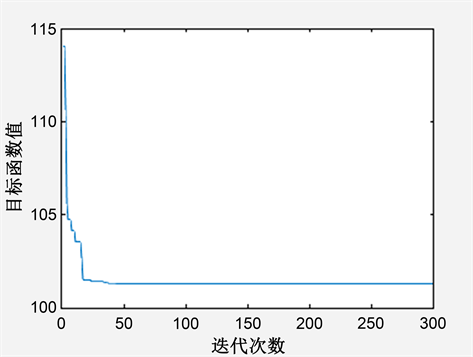﻿ 粒子群优化算法在工程中的应用

粒子群优化算法在工程中的应用An Application of Particle Swarm Optimization in Engineering

Abstract: In engineering design, optimization design finds the optimal solution from a wide variety of design methods, thereby improving the design quality. This paper studies the basic rationales of Particle Swarm Optimization (PSO) and analyzes its characteristics. We aim to reduce the weight of cover plate while maintaining its strength, stiffness and stability. After setting up a mathematical model of optimization design of cover board, we use PSO to solve the variables. Due to the advantage of combining both global search and local search, the application of PSO on design optimization of cover plate achieves a better result compared to several other constrained optimization methods.

1. 引言

2. 粒子群优化算法理论

${x}_{i}={\left(\begin{array}{cccc}{x}_{i1}& {x}_{i2}& \cdots & {x}_{id}\end{array}\right)}^{\text{T}},\text{\hspace{0.17em}}\text{\hspace{0.17em}}\text{\hspace{0.17em}}i=1,2,\cdots ,M$ (1)

${v}_{i}={\left(\begin{array}{cccc}{v}_{i1}& {v}_{i2}& \cdots & {v}_{id}\end{array}\right)}^{\text{T}},\text{\hspace{0.17em}}\text{\hspace{0.17em}}\text{\hspace{0.17em}}i=1,2,\cdots ,M$ (2)

$\begin{array}{l}{v}_{i}^{t+1}=w{v}_{i}^{t}+{c}_{1}{r}_{1}\left({p}_{i}^{t}-{x}_{i}^{t}\right)+{c}_{2}{r}_{2}\left({p}_{g}^{t}-{x}_{i}^{t}\right)\\ {x}_{i}^{t+1}={x}_{i}^{t}+{v}_{i}^{t}\end{array}$

${p}_{i}$ 为第i个粒子的历史最好解，即对应个体极值。

${p}_{g}^{t}=\mathrm{min}\left({p}_{i}^{t}\right)$，是所有粒子到t时刻为止求得的历史最优解。

w为惯性权重因子，为非负值，值的大小影响整体的寻优能力。

${c}_{1}$${c}_{2}$ 为加速因子，通常在0~2间取值。

${r}_{1}$${r}_{2}$ 为两个[0~1]之间变化的相对独立的随机均匀数。

(1) 首先初始化，按照种群规模大小，初始化每个粒子的位置、速度。

(2) 根据具体问题的目标函数，计算每个粒子的适应度，一般与目标函数值一致；

(3) 对于每个粒子，用它的适应度值和个体极值进行比较，求出每个粒子的个体最优值；

(4) 对于所有粒子，用适应度值和全局极值比较，求出全局极值；

(5) 根据公式(1) (2)更新各个粒子的速度及位置；

(6) 如果满足结束条件则输出结果，退出，否则返回(2)。

3. 粒子群算法在盖板优化中的应用Figure 1. Cover plate model

$I=2bt{\left(\frac{h}{2}\right)}^{2}=30t{h}^{2}$

${\tau }_{\mathrm{max}}=\frac{{Q}_{\mathrm{max}}}{2×0.5h}=\frac{180}{h}\left( MPa \right)$

${\sigma }_{\mathrm{max}}=\frac{{M}_{\mathrm{max}}}{I}×\frac{h}{2}=\frac{450}{th}\left( MPa \right)$

${\sigma }_{cr}=\frac{{\pi }^{2}E}{3\left(1-{\epsilon }^{2}\right)}{\left(\frac{t}{b}\right)}^{2}\approx 70{t}^{2}\left( MPa \right)$

${f}_{\mathrm{max}}=\frac{5q{l}^{4}}{384EI}=\frac{482}{th}$

$x=\left[\begin{array}{c}{x}_{1}\\ {x}_{2}\end{array}\right]=\left[\begin{array}{c}t\\ h\end{array}\right]$

$F\left(x\right)=2×60t+2×0.5h=120t+h=120{x}_{1}+{x}_{2}$

${g}_{1}\left(x\right)=-{x}_{1}<0$

${g}_{2}\left(x\right)=-{x}_{2}<0$

${g}_{3}\left(x\right)=1-\frac{\left[\tau \right]}{{\tau }_{\mathrm{max}}}=1-\frac{{x}_{2}}{4}\le 0$

${g}_{4}\left(x\right)=1-\frac{\left[\sigma \right]}{{\sigma }_{\mathrm{max}}}=1-\frac{7{x}_{1}{x}_{2}}{45}\le 0$

${g}_{5}\left(x\right)=1-\frac{{\sigma }_{cr}}{{\sigma }_{\mathrm{max}}}=1-\frac{7{x}_{1}^{3}{x}_{2}}{45}\le 0$

${g}_{6}\left(x\right)=1-\frac{1.5}{{f}_{\mathrm{max}}}=1-\frac{{x}_{1}{x}_{2}^{2}}{321}\le 0$Figure 2. Optimizing iterative processTable 1. Solutions of various optimization methods

4. 结束语

NOTES

*通讯作者。

 廖坤锐, 陈卫兵, 杨雪. 基于PCA和粒子群优化算法的焊点缺陷识别[J]. 计算机测量与控制, 2020, 28(5): 190-195.

 王芷馨, 王冬青, 韩增亮, 许崇立. 基于粒子群优化算法的非线性系统辨识[J]. 自动化与仪器仪表, 2020(5): 8-13.

 Guedria, N.B. (2016) Improved Accelerated PSO Algorithm for Mechanical Engineering Optimiza-tion Problems. Applied Soft Computing, 40, 455-467.
https://doi.org/10.1016/j.asoc.2015.10.048

 周树功, 李娟. 粒子群优化算法和支持向量机的上市公司信用风险预警[J]. 现代电子技术, 2020(43): 73-76.

 王晨, 王琪, 张萌. 粒子群优化神经网络的动力电池SOC估算研究[J]. 单片机嵌入式系统应用, 2020(6): 20-24.

 李旭贞. 基于粒子群算法的行星齿轮传动优化设计[J]. 机械工程与自动化, 2018, 211(6): 98-100.

 刘景森, 袁蒙蒙, 李煜. 基于改进樽海鞘群算法求解工程优化设计问题[J]. 系统仿真学报, 2020. http://kns.cnki.net/kcms/detail/11.3092.V.20200403.1115.005.html

 刘瑜, 马良. 量子蚂蚁算法及其在箱形盖板优化设计中的应用[J]. 机械设计与研究, 2009, 25(4): 56-58.

Top